# Feynman Diagrams homework help

• roam

## Homework Statement

For the following reactions draw Feynman diagrams, clearly labelling all the quarks, leptons and exchanged particles and stating the type of interaction involved (if more than one interaction could be involved give the most likely):

(i) ##\pi^0 \to \gamma + \gamma##

(ii) ##\bar{\Lambda} \to \bar{n}+\pi^0##

(iii) ##e^+ + e^- \to \nu_\tau + \bar{\nu_\tau}##

## The Attempt at a Solution

For (i) the pion decays into two photons. But the quark composition of ##\pi^0## is given by both ##u\bar{u}## and ##d\bar{d}##, so which one should I use in the diagram?Also I believe it decays by the weak interaction, but where exactly should I place the W- line on the diagram? Here's the diagram so far: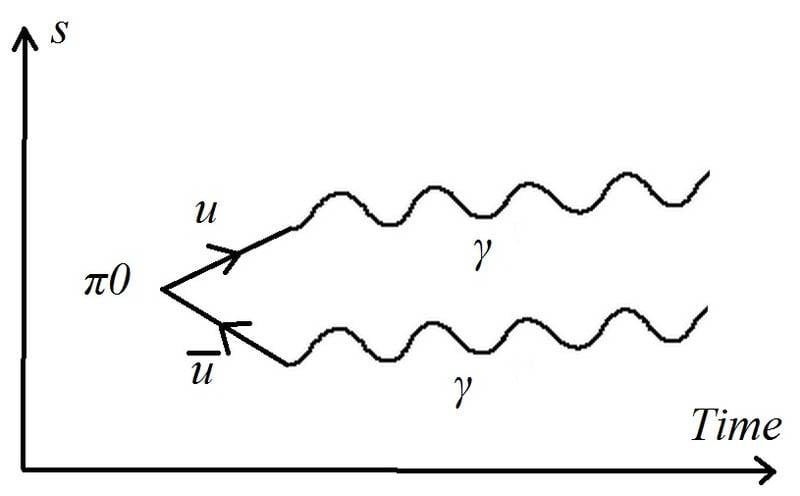(ii) Is ##\bar{\Lambda}## referring to the antiparticle of neutral lambda (##\Lambda^0##)? Here is my attempt: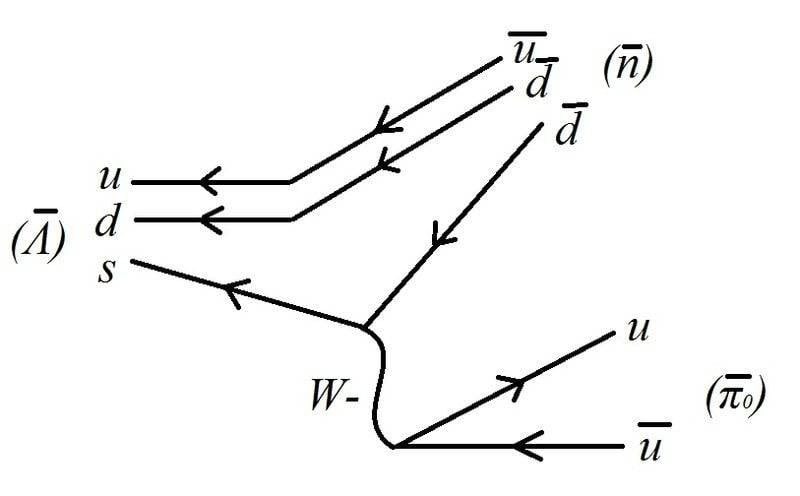Is this correct?

(iii) Is this the weak force since there are neutrinos involved? I'm not 100% sure if this is the electromagnetic or weak force. Here is my diagram: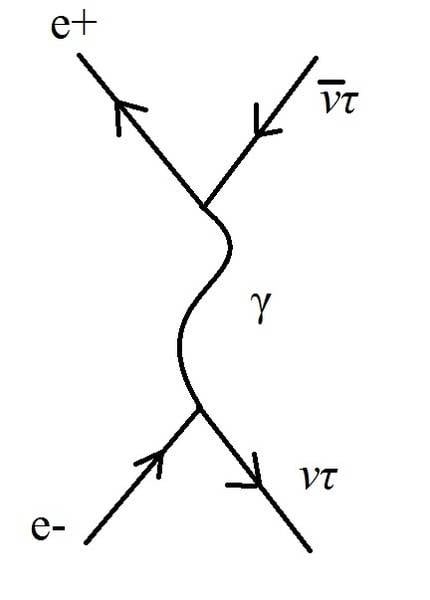(once again time flows horizontally)

Any help with these diagrams is greatly appreciated.

(i)
For (i) the pion decays into two photons. But the quark composition of ##\pi^0## is given by both ##u\bar{u}## and ##d\bar{d}##, so which one should I use in the diagram?Just use one of them, that is fine.

Your diagram does not work. A single quark cannot decay to a photon, and the pion is not a single vertex where suddenly two quarks emerge out of nothing.
This is not a weak decay.

(ii)
##\bar{\Lambda}## is the antiparticle of the neutral baryon Λ, yes.

The diagram is not correct - the W is charged, you try to make a neutral particle coming out of a charged particle! The W only couples to "one up-type quark" plus "one down-type quark" in the vertices. The strange quark is "down-type", so the other quark at this side (where you used a down) has to be an up-quark.
And don't forget the antiquark signs for the initial lambda.

(iii)
Is this the weak force since there are neutrinos involved?
If neutrinos are produced (or annihilated), the weak force is always part of the interaction, yes.
Your Feynman diagram violates charge conservation at both vertices - the charge of the lepton "vanishes", that is not possible.

•1 person
Thank you so much for the very helpful post.

(ii) Here is what I did: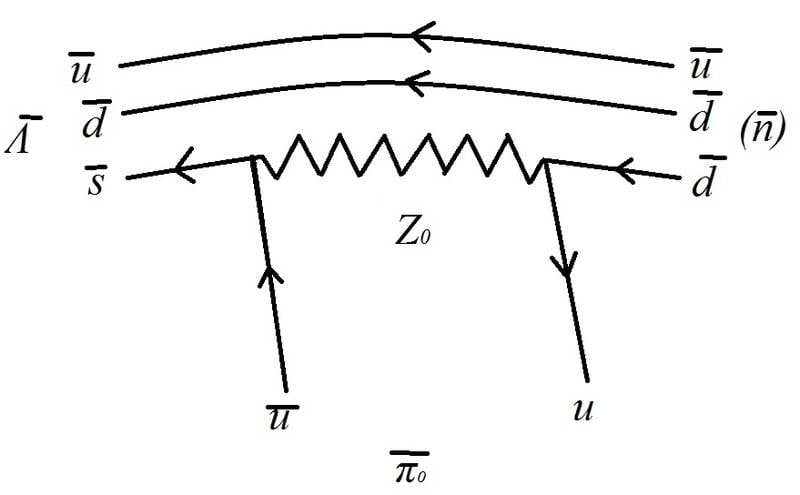is this correct now? (I used the Z0 boson as the exchange particle)(iii) This is the weak interaction. So emission of W- (or absorption of W+) should now conserve charge: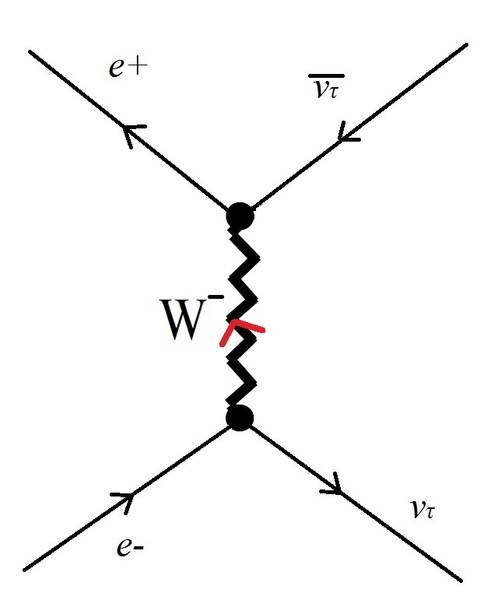I'm a little bit confused. In this reaction shouldn't we get the corresponding electron neutrino (##\nu_e##) instead of tau neutrino? I thought usually for a lepton l-, we have ##l^- \to \nu_l + W^-##.

(i) Is this the electromagnetic interaction? Would it be correct to join the two quarks? I mean like the following: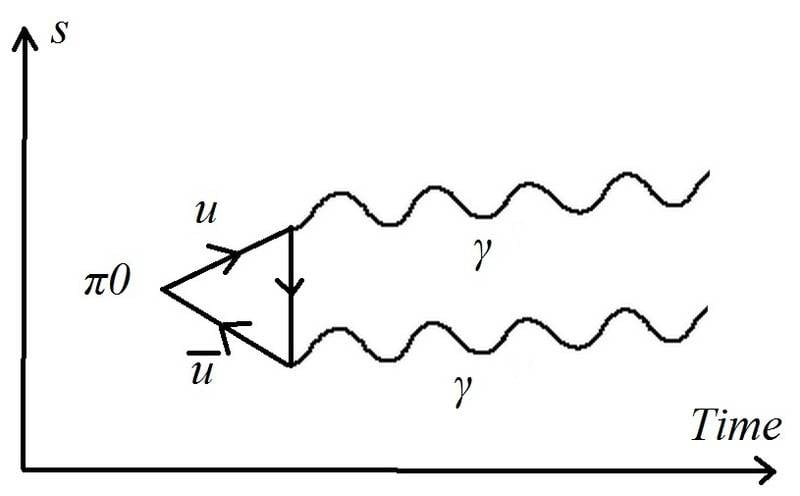Thanks.

Last edited:
Your diagram for (i) looks ok now.

(ii) This does not work. The Z couplings do not break quark flavor and must be connected to quarks of the same flavor. In addition, charge is not conserved in your vertices.

(iii) The W does not break lepton flavor so it cannot be in a vertex together with an electron and a tau neutrino. In fact, this is the defining property of neutrino flavor. The diagram must be different.

(ii) This does not work. The Z couplings do not break quark flavor and must be connected to quarks of the same flavor. In addition, charge is not conserved in your vertices.

So how else could you do (ii)? Are you saying I should use W- instead?

Because at one vertex we have a charge of +1/3-2/3=-1/3, and at the other one we have 1/3+2/3=1.

(iii) The W does not break lepton flavor so it cannot be in a vertex together with an electron and a tau neutrino. In fact, this is the defining property of neutrino flavor. The diagram must be different.

In what way should it be different? I'm confused and I can't think of any other way of drawing it. It would make more sense to me if you could describe how it should look like, at least maybe in a different context or a similar example.

Edit: I understand that, for example, e- converts to ##\nu_e## with emission of W-, but e- never goes to ##\nu_{tau/ \mu}## (a different lepton flavour). But reaction in (iii) involves weak interaction, so I'm not sure how else to use the W boson in the diagram.

Last edited:
(ii) What is the charge of the ##\bar s## and the ##\bar u##? What must then be the charge of the exchanged particle? Think about what is going into the vertices and what is going out and write down charge conservation as "in = out".

(iii) To draw the diagram for you would be against forum rules. I can only give you hints: You have already realized it should be a weak decay, that is correct. What vertices involving charged leptons and neutrinos do you have available in weak interactions (i.e., with W and Z bosons)?

(ii) What is the charge of the ##\bar s## and the ##\bar u##? What must then be the charge of the exchanged particle? Think about what is going into the vertices and what is going out and write down charge conservation as "in = out".

Charges for ##\bar s## and the ##\bar u## are +1/3e and -2/3e respectively. So the difference in charge would be -1/3. So we can't use W boson because it has a charge of -1?(iii) To draw the diagram for you would be against forum rules. I can only give you hints: You have already realized it should be a weak decay, that is correct. What vertices involving charged leptons and neutrinos do you have available in weak interactions (i.e., with W and Z bosons)?

It's easier to understand this visually but I'm not asking for a diagram, a more clear hint is appreciated since I've already shown my attempt several times.

I have two vertices available one with a positron and an anti tau neutrino, and another vertex with an electron and a tau neutrino. I understand the problem with change of lepton flavour but I'm not sure how to resolve it.

Charges for ##\bar s## and the ##\bar u## are +1/3e and -2/3e respectively. So the difference in charge would be -1/3. So we can't use W boson because it has a charge of -1?What charges are going into the vertex and which are going out?

It's easier to understand this visually but I'm not asking for a diagram, a more clear hint is appreciated since I've already shown my attempt several times.

I have two vertices available one with a positron and an anti tau neutrino, and another vertex with an electron and a tau neutrino. I understand the problem with change of lepton flavour but I'm not sure how to resolve it.

Since you cannot have a vertex with a W together with an electron and a tau neutrino, this would suggest that the W is not the mediator. What other possibilities are there?

What charges are going into the vertex and which are going out?

Oops, yes -2/3 is going in and +1/3 is going out, so there is a difference of +1. An absorption of W+ (or emission of W-) works for this vertex. But for the vertex on the other side, there is a +1/3 going in and +2/3 coming out, so how does that work out?

Since you cannot have a vertex with a W together with an electron and a tau neutrino, this would suggest that the W is not the mediator. What other possibilities are there?

That's true but W and Z are the only mediators of the weak force. If we use Z0 it violates charge conservation and also lepton flavour.

Oops, yes -2/3 is going in and +1/3 is going out, so there is a difference of +1. An absorption of W+ (or emission of W-) works for this vertex. But for the vertex on the other side, there is a +1/3 going in and +2/3 coming out, so how does that work out?

On the left side, you have an incoming ##\bar s## and an outgoing ##\bar u## and an outgoing ##W##. On the right side the ##W## is incoming and the quarks outgoing. Look from left to right when determining this. Alternatively you can go through the fermion flow lines, but then the flow is always of a particle in the direction of the flow line and an antiparticle if you go against the flow line.

That's true but W and Z are the only mediators of the weak force. If we use Z0 it violates charge conservation and also lepton flavour.

Yes, if you use the Z in the way shown in your diagram it violates both, so we cannot use it in this way. Is there another way of using the Z? That is, can you think of another way of connecting the particles with the mediator?

•1 person
Yes, if you use the Z in the way shown in your diagram it violates both, so we cannot use it in this way. Is there another way of using the Z? That is, can you think of another way of connecting the particles with the mediator?

Would it be correct to place the electron and positron coming in and out of one vertex, and the neutrino and anti-neutrino at the other vertex (the two vertices are joined by Z0)?

Yes, in this way both vertices preserve both charge and lepton flavor.

Thank you so much for your help, I really appreciate it.

Just a remark to (i): let both quarks for the pion come in as parallel lines, similar to the diagram for the lambda.

•1 person
Thank you for the tip, I'll do that!